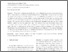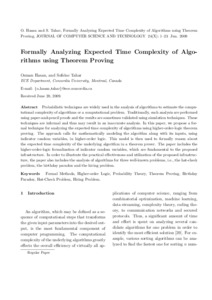Title:

# Formally Analyzing Expected Time Complexity of Algorithms Using Theorem Proving

Hasan, Osman and Tahar, Sofiène (2010) Formally Analyzing Expected Time Complexity of Algorithms Using Theorem Proving. Journal of Computer Science and Technology, 25 (6). pp. 1305-1320. ISSN 1000-9000Preview
Text (application/pdf)
formally_analyzing_expected_time.pdf - Accepted Version
361kB

Official URL: http://dx.doi.org/10.1007/s11390-010-9407-0

## Abstract

Probabilistic techniques are widely used in the analysis of algorithms to estimate the computational complexity of algorithms or a computational problem. Traditionally, such analyses are performed using paper-and-pencil proofs and the results are sometimes validated using simulation techniques. These techniques are informal and thus may result in an inaccurate analysis. In this paper, we propose a formal technique for analyzing the expected time complexity of algorithms using higher-order-logic theorem proving. The approach calls for mathematically modeling the algorithm along with its inputs, using indicator random variables, in higher-order logic. This model is then used to formally reason about the expected time complexity of the underlying algorithm in a theorem prover. The paper includes the higher-order-logic formalization of indicator random variables, which are fundamental to the proposed infrastructure. In order to illustrate the practical effectiveness and utilization of the proposed infrastructure, the paper also includes the analysis of algorithms for three well-known problems, i.e., the hat-check problem, the birthday paradox and the hiring problem.

Divisions: Concordia University > Gina Cody School of Engineering and Computer Science > Electrical and Computer Engineering Article Yes Hasan, Osman and Tahar, Sofiène Journal of Computer Science and Technology 2010 10.1007/s11390-010-9407-0 974502 ANDREA MURRAY 31 Jul 2012 20:11 18 Jan 2018 17:38
All items in Spectrum are protected by copyright, with all rights reserved. The use of items is governed by Spectrum's terms of access.

Repository Staff Only: item control pageResearch related to the current document (at the CORE website)
Back to top